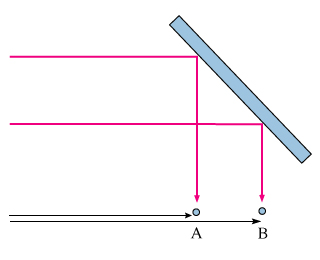###### FREE Expert Solution

Part A

At point A, there is destructive interference.

Destructive interference is expressed as:

$\overline{){{\mathbf{x}}}_{{\mathbf{A}}}{\mathbf{=}}{\mathbf{\left(}}{\mathbf{n}}{\mathbf{+}}\frac{\mathbf{1}}{\mathbf{2}}{\mathbf{\right)}}{\mathbf{\lambda }}}$

At point B, there is constructive interference, expressed as:

$\overline{){{\mathbf{x}}}_{{\mathbf{B}}}{\mathbf{=}}{\mathbf{n}}{\mathbf{\lambda }}}$

97% (316 ratings)###### Problem Details

You are listening to the FM radio in your car. As you come to a stop at a traffic light, you notice that the radio signal is fuzzy. By pulling up a short distance, you can make the reception clear again. In this problem, we work through a simple model of what is happening.

• the direct route from the transmitter
• an indirect route via reflection off a building

Because the two paths have different lengths, they can constructively or destructively interfere. Assume that the transmitter is very far away, and that the building is at an a 45° angle from the path to the transmitter.

Point A in the figure is where you originally stopped, and point B is where the station is completely clear again. Finally, assume that the signal is at its worst at point A, and at its clearest at point B.Part A

What is the distance d between points A and B?

Part B

Your FM station has a frequency of 100 megahertz. The speed of light is about 3.00x108 meters per second. What is the distance d between points A and B?